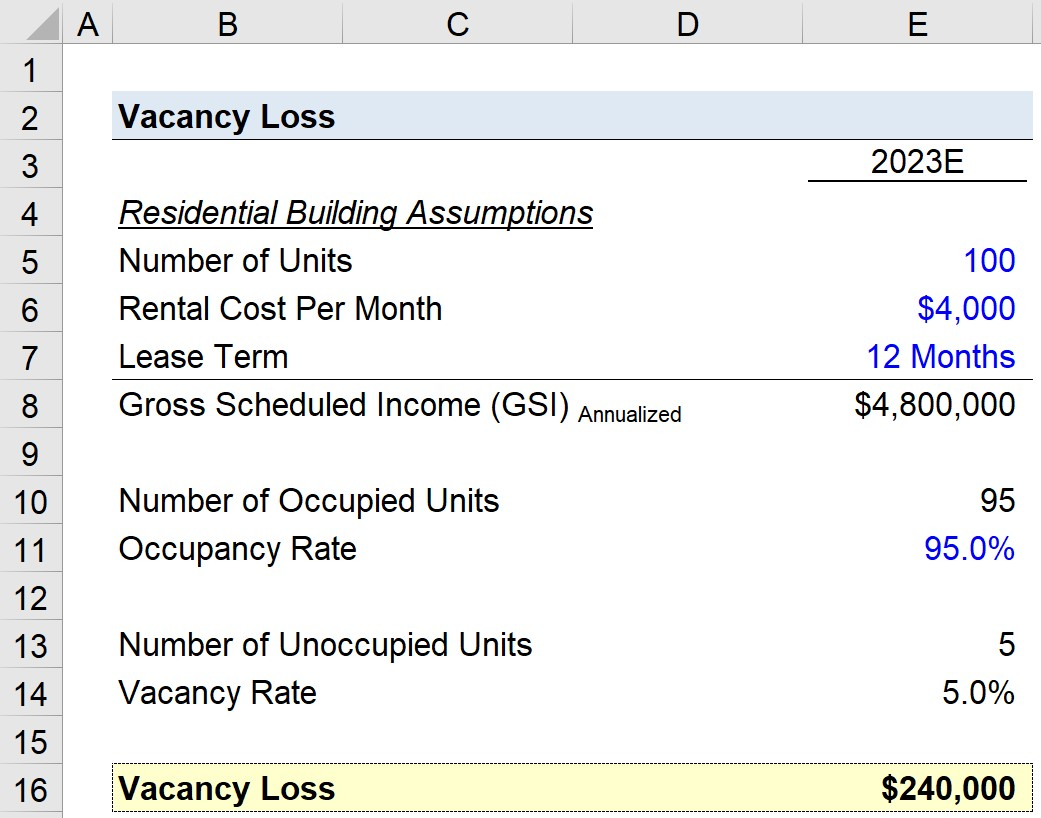Welcome to Wall Street Prep! Use code at checkout for 15% off.# Vacancy Loss

Guide to Understanding Vacancy Loss## How to Calculate Vacancy Loss?

The vacancy loss refers to the dollar amount of rental income lost because of unoccupied units, where there are no tenants.

While there is a negative connotation, the vacancy loss metric can also be viewed as representing the potential rental income that could be earned in the future.

The process of calculating the real estate metric involves multiplying the vacancy assumption by the gross potential income generated by the property, i.e. the rental income if all units were occupied.

The resulting amount is the rental income lost by the unoccupied units.

When projecting the anticipated loss, assumptions are necessary regarding the conditions of the real estate market, tenant demand, property conditions (i.e. number of available space vs. unavailable space due to construction), and retention of existing tenants.

For property owners striving to reduce their vacancy loss, the following measures can be taken:

• Offer Incentives, e.g. Free Months
• Reduction to Rent, i.e. Net Effective Rent < Gross Rent
• Interior Improvements and Renovations## Vacancy Loss Formula

The formula for calculating vacancy loss is as follows.

Vacancy Loss = Gross Scheduled Income (GSI) × Vacancy Rate

Where:

• Gross Scheduled Income (GSI) → The gross scheduled income is the total amount of potential rental income that could be generated by commercial property, assuming the property is at full capacity, i.e. 100% occupancy.
• Vacancy Rate → The vacancy rate is the implied percentage of unoccupied units, and can be computed as one minus the occupancy rate.

## Vacancy Loss Calculator

We’ll now move to a modeling exercise, which you can access by filling out the form below.#### Excel TemplateSubmitting...

## Vacancy Loss Calculation Example

Suppose the property manager of a residential building is attempting to determine the expected vacancy loss in anticipation of the upcoming year, 2023.

The residential building has 100 units available for rent in total, with each unit priced at the same monthly rate of \$4,000.

While an unrealistic scenario – for purposes of this exercise – we’ll assume that all rental lease commitments are on a 12-month basis.

• Number of Units = 100
• Rental Cost Per Month = \$4,000
• Lease Term = 12 Months

Given those assumptions, we can calculate the gross scheduled income (GSI) by multiplying all three assumptions.

• Gross Scheduled Income (GSI) = 100 × \$4,000 × 12 Months = \$4,800,000

The \$4.8 million represents the total potential rental income assuming 100% occupancy, as well as no concessions or discounts that affect the net effective rent paid by tenants.

Next, we’ll assume that the occupancy rate as of the present date is 95%, meaning that 95 units have an existing tenant that has signed a lease and will be obligated to pay rent each month.

The vacancy rate equals one minus the occupancy rate, so the vacancy rate is 5.0%.

• Occupancy Rate = 95%
• Vacancy Rate = 1 – 95% = 5.0%
• Occupied Units = 95 Units
• Unoccupied Units = 5 Units

By multiplying the gross scheduled income (GSI) by the vacancy rate, we arrive at a vacancy loss of \$240,000, which represents the rental income expected to be lost in 2023 unless those unoccupied units are filled.

• Vacancy Loss = \$4,800,000 × 5.0% = \$240,00020+ Hours of Online Video Training

### Master Real Estate Financial Modeling

This program breaks down everything you need to build and interpret real estate finance models. Used at the world's leading real estate private equity firms and academic institutions.

Inline Feedbacks